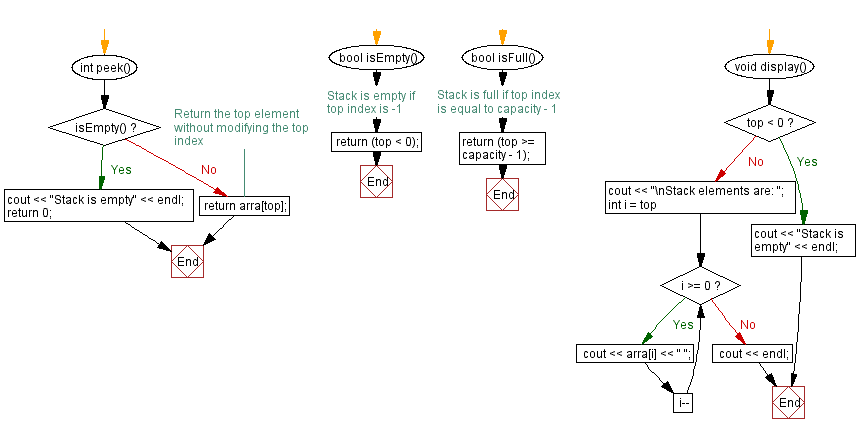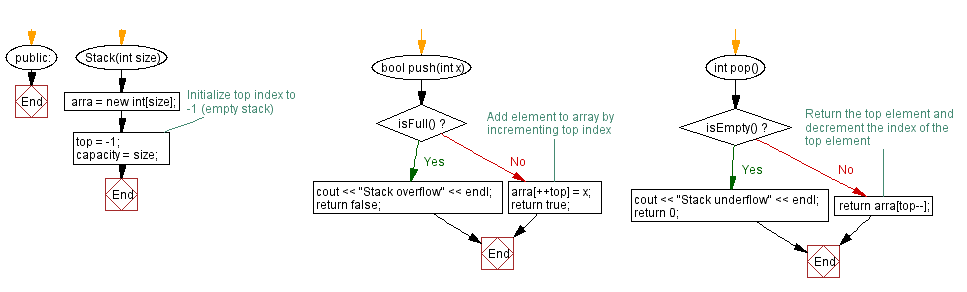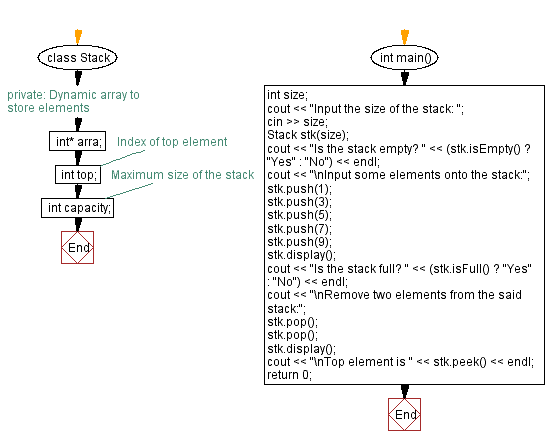﻿ C++ Implement a stack using a dynamic array with push, pop

# C++ Stack Exercises: Implement a stack using a dynamic array with push, pop

## C++ Stack: Exercise-20 with Solution

Write a C++ program to implement a stack using a dynamic array with push and pop operations. Find the top element of the stack and check if the stack is empty or not.

Test Data:
Input the size of the stack: Is the stack empty? Yes
Input some elements onto the stack:
Stack elements are: 9 7 5 3 1
Is the stack full? No

Sample Solution:

C++ Code:

``````#include <iostream>

using namespace std;

class Stack {
private:
// Dynamic array to store elements
int* arra;
int top;        // Index of top element
int capacity;   // Maximum size of the stack
public:
Stack(int size) {
arra = new int[size];
top = -1;       // Initialize top index to -1 (empty stack)
capacity = size;
}

bool push(int x) {
if (isFull()) {
cout << "Stack overflow" << endl;
return false;
}
//Add element to array by incrementing top index
arra[++top] = x;
return true;
}

int pop() {
if (isEmpty()) {
cout << "Stack underflow" << endl;
return 0;
}
//Return the top element and decrement the index of the top element
return arra[top--];
}

int peek() {
if (isEmpty()) {
cout << "Stack is empty" << endl;
return 0;
}
// Return the top element without modifying the top index
return arra[top];
}

bool isEmpty() {
// Stack is empty if top index is -1
return (top < 0);
}

bool isFull() {
// Stack is full if top index is equal to capacity - 1
return (top >= capacity - 1);
}

void display() {
if (top < 0) {
cout << "Stack is empty" << endl;
return;
}
cout << "\nStack elements are: ";
for (int i = top; i >= 0; i--)
cout << arra[i] << " ";
cout << endl;
}
};

int main() {
int size;
cout << "Input the size of the stack: ";
cin << size;
Stack stk(size);
cout << "Is the stack empty? " << (stk.isEmpty() ? "Yes" : "No") << endl;
cout << "\nInput some elements onto the stack:";
stk.push(1);
stk.push(3);
stk.push(5);
stk.push(7);
stk.push(9);
stk.display();
cout << "Is the stack full? " << (stk.isFull() ? "Yes" : "No") << endl;
cout << "\nRemove two elements from the said stack:";
stk.pop();
stk.pop();
stk.display();
cout << "\nTop element is " << stk.peek() << endl;
return 0;
}
``````

Sample Output:

```Input the size of the stack: 8
Is the stack empty? Yes

Input some elements onto the stack:
Stack elements are: 9 7 5 3 1
Is the stack full? No

Remove two elements from the said stack:
Stack elements are: 5 3 1

Top element is 5
```

Flowchart:CPP Code Editor: